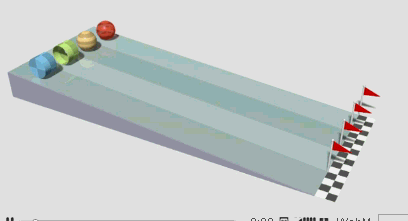## Moment of Inertia Calculator

 Enter the mass of hollow material : (lbm,kg) Distance between axis and rotation : (ft,m) Moment of inertia I:

Moment of inertia depends on the shape of the body and If the axes of rotation is different, the moment of inertia is different.

The diagram below shows four objects racing down a plane. Their shape are different, we could know they have different moment of inertia.### Moment of inertia Formula

Moment of inertia = M * D2

Where D = Distance between axis and rotation, M = Angular Mass of the Hollow material.

For example, When Distance between axis and rotation is 1.2m, the mass of hollow material is 2 kg, then Moment of inertia I is 2.88.

Thinkcalculator.com provides you helpful and handy calculator resources.